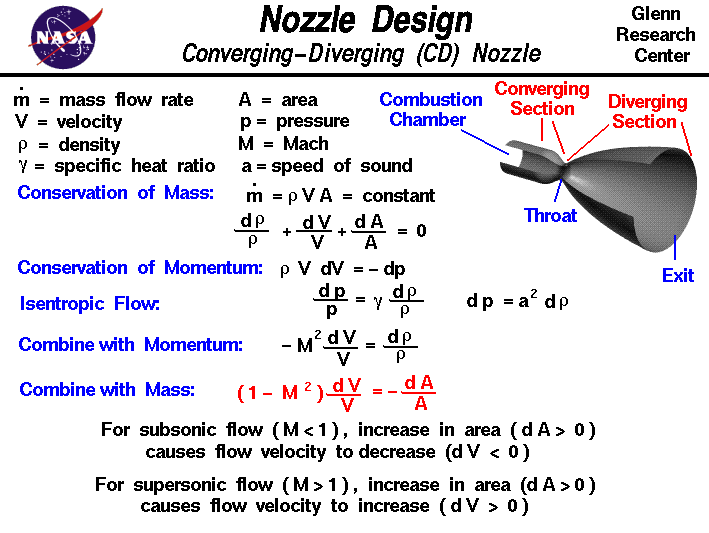+ Text Only Site
+ Non-Flash Version
+ Contact GlennRamjets, scramjets, and rockets all use nozzles to accelerate hot exhaust to produce thrust as described by Newton's third law of motion. The amount of thrust produced by the engine depends on the mass flow rate through the engine, the exit velocity of the flow, and the pressure at the exit of the engine. The value of these three flow variables are all determined by the nozzle design. A nozzle is a relatively simple device, just a specially shaped tube through which hot gases flow. Ramjets and rockets typically use a fixed convergent section followed by a fixed divergent section for the design of the nozzle. This nozzle configuration is called a convergent-divergent, or CD, nozzle. In a CD nozzle, the hot exhaust leaves the combustion chamber and converges down to the minimum area, or throat, of the nozzle. The throat size is chosen to choke the flow and set the mass flow rate through the system. The flow in the throat is sonic which means the Mach number is equal to one in the throat. Downstream of the throat, the geometry diverges and the flow is isentropically expanded to a supersonic Mach number that depends on the area ratio of the exit to the throat. The expansion of a supersonic flow causes the static pressure and temperature to decrease from the throat to the exit, so the amount of the expansion also determines the exit pressure and temperature. The exit temperature determines the exit speed of sound, which determines the exit velocity. The exit velocity, pressure, and mass flow through the nozzle determines the amount of thrust produced by the nozzle. On this slide we derive the equations which explain and describe why a supersonic flow accelerates in the divergent section of the nozzle while a subsonic flow decelerates in a divergent duct. We begin with the conservation of mass equation: mdot = r * V * A = constant where mdot is the mass flow rate, r is the gas density, V is the gas velocity, and A is the cross-sectional flow area. If we differentiate this equation, we obtain: V * A * dr + r * A * dV + r * V * dA = 0 divide by (r * V * A) to get: dr / r + dV / V + dA / A = 0 Now we use the conservation of momentum equation: r * V * dV = - dp and an isentropic flow relation: dp / p = gam * dr / r where gam is the ratio of specific heats. This is Equation #10 on the page which contains the derivation of the isentropic flow relations We can use algebra on this equation to obtain: dp = gam * p / r * dr and use the equation of state p / r = R * T where R is the gas constant and T is temperature, to get: dp = gam * R * T * dr gam * R * T is the square of the speed of sound a: dp = (a^2) * dr Combining this equation for the change in pressure with the momentum equation we obtain: r * V * dV = - (a^2) * dr V / (a^2) * dV = - dr / r - (M^2) * dV / V = dr / r using the definition of the Mach number M = V / a. Now we substitute this value of (dr /r) into the mass flow equation to get: - (M^2) * dV / V + dV / V + dA / A = 0 (1 - M^2) * dV / V = - dA / A This equation tells us how the velocity V changes when the area A changes, and the results depend on the Mach number M of the flow. If the flow is subsonic then (M < 1) and the term multiplying the velocity change is positive (1 - M^2 > 0). An increase in the area (dA > 0 ) produces a negative increase (decrease) in the velocity (dV < 0). For our CD nozzle, if the flow in the throat is subsonic, the flow downstream of the throat will decelerate and stay subsonic. So if the converging section is too large and does not choke the flow in the throat, the exit velocity is very slow and doesn't produce much thrust. On the other hand, if the converging section is small enough so that the flow chokes in the throat, then a slight increase in area causes the flow to go supersonic. For a supersonic flow (M > 1) the term multiplying velocity change is negative (1 - M^2 < 0). Then an increase in the area (dA > 0) produces an increase in the velocity (dV > 0). This effect is exactly the opposite of what happens subsonically. Why the big difference? Because, to conserve mass in a supersonic (compressible) flow, both the density and the velocity are changing as we change the area. For subsonic (incompressible) flows, the density remains fairly constant, so the increase in area produces only a change in velocity. But in supersonic flows, there are two changes; the velocity and the density. The equation: - (M^2) * dV / V = dr / r tells us that for M > 1, the change in density is much greater than the change in velocity. To conserve both mass and momentum in a supersonic flow, the velocity increases and the density decreases as the area is increased. Activities: Guided Tours Navigation ..Beginner's Guide Home Page+ Inspector General Hotline + Equal Employment Opportunity Data Posted Pursuant to the No Fear Act + Budgets, Strategic Plans and Accountability Reports + Freedom of Information Act + The President's Management Agenda + NASA Privacy Statement, Disclaimer, and Accessibility CertificationEditor: Tom Benson NASA Official: Tom Benson Last Updated: May 07 2021 + Contact Glenn